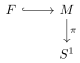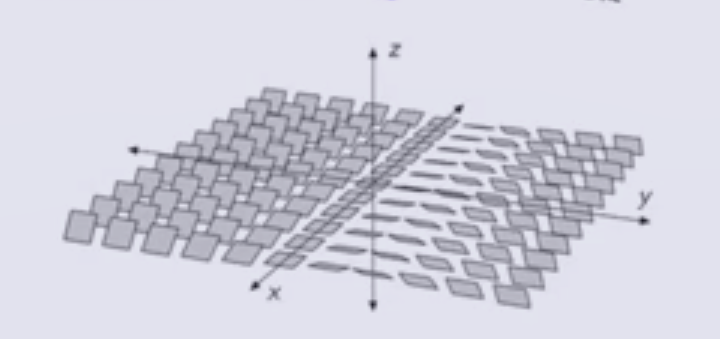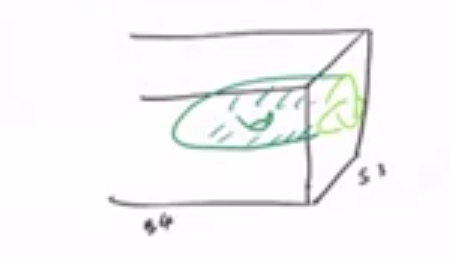# 1 Lecture 1: Overview (Wednesday, January 13)

## 1.1 Course Logistics

Note (DZG): Everything in this section comes from Akram!

### 1.1.1 Description

“I am teaching a topics course about Heegaard Floer homology next semester. Heegaard Floer homology was defined by Peter Ozsváth and Zoltan Szabó around 2000. It is a package of powerful invariants of smooth 3- and 4-manifolds, knots/links and contact structures. Over the last two decades, it has become a central tool in low-dimensional topology. It has been used extensively to study and resolve important questions concerning unknotting number, slice genus, knot concordance and Dehn surgery. It has been employed in critical ways to study taut foliations, contact structures and smooth 4-manifolds. There are also many rich connections between Heegaard Floer homology and other manifold and knot invariants coming from gauge theory as well as representation theory. We will learn the basic construction of Heegaard Floer homology, starting with the definition of the 3-manifold and knot invariants. In the second half of this course, we will turn to computations and applications of the theory to low-dimensional topology and knot theory. In particular, several numerical invariants have been defined using this homological invariants. At the end of the semester, I would expect each one of you to learn the construction of one of these invariants (of course with my help) and present it to the class.”

### 1.1.3 Research Papers

• [OSz04a?] Peter Ozsváth and Zoltán Szabó, Holomorphic disks and topological invariants for closed three-manifolds. Ann. of Math. (2) 159 (2004), no. 3, 1027–1158. arXiv:math/0101206
• [OSz04b?] Peter Ozsváth and Zoltán Szabó, Holomorphic disks and three-manifold invariants: properties and applications. Ann. of Math. (2) 159 (2004), no. 3, 1159–1245. arXiv:math/0105202
• [OSz04c?] Peter Ozsváth and Zoltán Szabó, Holomorphic disks and knot invariants. Adv. Math. 186 (2004), no. 1, 58–116. arXiv:math/0209056
• [OSz06?] Peter Ozsváth and Zoltán Szabó, Holomorphic triangles and invariants for smooth four manifolds. Adv. Math. 202 (2006), no. 2, 326–400. arXiv:math/0110169
• [Per08?] Timothy Perutz, Hamiltonian handleslides for Heegaard Floer homology. Proceedings of Gökova Geometry-Topology Conference 2007, 15–35, Gökova Geometry/Topology Conference (GGT), Gökova, 2008. arXiv:0801.0564

## 1.2 Intro and Motivation

We’ll assume everything is smooth and oriented.

To closed 3-manifolds $$M$$ we can assign a graded abelian group $$\widehat{HF}(M)$$, which can be computed combinatorially1 . There are several variants:

• $$HF^- \in {\mathsf{grMod}}({\mathbb{Z}}_2[u])$$,2

• $$HF^+ \in {\mathsf{Mod}}({\mathbb{Z}}_2[u, u ^{-1} ] / u {\mathbb{Z}}_2[u])$$.

• $$HF^\infty \in {\mathsf{grMod}}({\mathbb{Z}}_2[u, u ^{-1} ])$$,

$$HF^+$$ and $$HF^\infty$$ can be computed using $$HF^-$$. In general, we’ll write $$HF^{-}$$ to denote constructions that work with any of the above variants.

Note that $${\mathbb{Z}}_2$$ can be replaced with $${\mathbb{Z}}$$, but it’s technical and we won’t discuss it here. For the first half of the course, we’ll just discuss $$\widehat{HF}$$, and we’ll discuss the latter 3 in the second half.

## 1.3 Geometric Information

These invariants can be used to compute the Thurston seminorm of a 3-manifold:

A homology class $$\alpha\in H_2(M)$$ can be represented as $$\alpha\in [S]$$ for $$S$$ a closed surface whose fundamental class represents $$\alpha$$ where $$S = \bigcup_{i=1}^n S_i$$ can be a union of closed embedded surfaces $$S_i$$. Then we first compute \begin{align*} \max\left\{{0, - \chi(S_i) }\right\} = \begin{cases} 0 & \text{if } S_i \cong {\mathbb{S}}^2, {\mathbb{T}}^2 \\ \\ - \chi(S_i) = 2g(S_i) - 2 & \text{ else} . \end{cases} .\end{align*} Note that the max checks if $$\chi$$ is positive. Then define \begin{align*} {\left\lVert { \alpha } \right\rVert} \mathrel{\vcenter{:}}=\min_S \qty{ \sum_{i=1}^n \max\left\{{0, - \chi(S_i) }\right\} } ,\end{align*} where we sum over the embedded subsurfaces and check which overall surface gives the smallest norm.

Note that this can’t be a norm, since if $${\mathbb{S}}^2, {\mathbb{T}}^2 \in [S] \implies {\left\lVert {\alpha } \right\rVert}= 0$$.

$$HF$$ detects3 the Thurston seminorm, and there is a splitting as groups/modules \begin{align*} HF^{-}(M) = \bigoplus _{\mathfrak{s} \in {\operatorname{Spin}}^c(M)} HF^{-}(M, S) \end{align*} where $$S \in {\operatorname{Spin}}^c(M)$$ is a spin$$^c$$ structure: an oriented 2-dimensional vector bundle on $$M$$ (up to some equivalence).

The Thurston norm $${\left\lVert {a} \right\rVert}$$ can be computed from this data by considering a perturbed version of $$\widehat{HF}$$, denoted $$\underline{\widehat{HF}}$$, in the following way: taking the first Chern class $$c_1(\mathfrak{s}) \in H^2(M)$$ (which can be associated to every 2-dimensional vector bundle), we have \begin{align*} {\left\lVert { \alpha} \right\rVert} = \max_{\underline{\widehat{HF}}(M, \mathfrak{s} ) \neq 0 } {\left\lvert {{\left\langle { c_1(\mathfrak{s}) },~{ \alpha } \right\rangle} } \right\rvert} .\end{align*}

Floer homology groups split over these spin$$^c$$ structures and can be used to compute Thurston norms.

Given $$F \subseteq M$$ with genus $$g\geq 2$$, $$HF$$ detects if $$M$$ fibers over $$S^1$$ with $$F$$ as a fiber, i.e. there exists a fiber bundleThis uses the existence of the splitting over spin$$^c$$ structures and uses $$HF^+$$ in the following way: such a bundles exists if and only if \begin{align*} \bigoplus _{{\left\langle { c_1(\mathfrak{s}) },~{ [F] } \right\rangle} =2g-2} HF^+(M, \mathfrak{s}) = {\mathbb{Z}} .\end{align*}

Equivalently,

• A smooth oriented nowhere integrable 2-plane field $$\xi$$, or

• A 2-plane field $$\xi \mathrel{\vcenter{:}}=\ker( \alpha)$$ where $$\alpha$$ is a 1-form such that $$\alpha\wedge d \alpha > 0$$.4

The standard contact structure on $${\mathbb{R}}^3$$ is given by \begin{align*} \alpha\mathrel{\vcenter{:}}= dz - ydz ,\end{align*} which yields the following 2-plane field $$\xi \mathrel{\vcenter{:}}=\ker \alpha$$:2-Plane Field in $${\mathbb{R}}^3$$

You can see that $$z=0 \implies y=0$$, so the $$xy{\hbox{-}}$$plane is in the kernel, yielding the flat planes down the middle:

To each such $$\xi$$ one can associate a contact class $$c(\xi) \in \widehat{HF}(-M)$$, where $$-M$$ is $$M$$ with the reversed orientation.

This gives obstructions for two of the following important properties of contact structures:

• Being overtwisted, or
• Being Stein fillable.
• If $$\xi$$ is overtwisted, then $$c(\xi) = 0$$.
• If $$\xi$$ is Stein fillable, then $$c(\xi) \neq 0$$.

We’ll also discuss similar invariants for knots that were created after these invariants for manifolds.

Recall that a knot is an embedding $$S^1 \hookrightarrow M$$.

Given a knot $$K \subseteq M$$ a 3-manifold (e.g. $$M = S^3$$), there is extra algebraic structure on $$\widehat{CF}(M)$$: a filtration. These allow defining a new bigraded abelian group $$\widehat{HFK}(M, K)$$ (which is also a $${\mathbb{Z}}_2{\hbox{-}}$$vector space) that takes includes the information of $$K$$. This yields a decomposition \begin{align*} \widehat{HFK}(M, K) = \bigoplus _{m, a} \widehat{HFK}_m(M, K, a) .\end{align*}

This similarly works for other variants: there is a filtration on $$CF^-(M)$$ which yields $$HFK^-(M, K)$$, a bigraded $${\mathbb{Z}}_2[u]{\hbox{-}}$$module.

### 1.3.1 Some properties of Knot Floer Homology

$$\widehat{HFK}(K)$$ categorifies the Alexander polynomial $$\Delta_K(t)$$ of $$K$$, i.e. taking the graded Euler characteristic yields \begin{align*} \Delta_K (t) =\sum_{m, a} (-1)^m\qty{ \dim \widehat{HFK}_m(K, a) } t^a .\end{align*}

$$\widehat{HFK}(K)$$ detects the Seifert genus of a knot $$g(K)$$, defined as the smallest $$g$$ such that there exists an embedded surface5 $$F$$ of genus $$g$$ in $$S^3$$ that bounds $$K$$, so $${{\partial}}F = K$$.

The unknot bounds a disc, so its genus is zero:

Using the “outside” disc on the trefoil, find 3 bands that show its genus is 1.

The genus can be computed by setting $$\widehat{HFK}(K, a) \mathrel{\vcenter{:}}=\bigoplus _m \widehat{HFK}_m(K, a)$$, which yields \begin{align*} g(k) = \max \left\{{ a {~\mathrel{\Big|}~}\widehat{HFK}(K, a) \neq 0 }\right\} .\end{align*} Note that the $$a$$ grading here is referred to as the Alexander grading.

$$\widehat{HFK}$$ detects whether or not a knot is fibered, where $$K$$ is fibered if and only if it admits an $$S^1$$ family $$F_t$$ of Seifert surfaces such that $$t\neq s\in S^1 \implies F_t \cap F_s = K$$. I.e., there is a fibration on the knot complement where each fiber is a Seifert surface:The unknot is fibered by $${\mathbb{D}}^2$$s:

This is “detected” in the following sense: $$K$$ is fibered if and only if \begin{align*} \widehat{HFK}(k, g(K)) = {\mathbb{Z}}_2 .\end{align*}

Let $$K \subseteq S^3$$. We know $$S^3 = {{\partial}}B^4$$, so we consider all of the smoothly properly embedded surfaces $$F$$ in $$B^4$$ such that $${{\partial}}F = K$$ and take the smallest genus:Knot in $$S^3$$ bounding a surface in $$B^4$$

We thus define the slice genus or 4-ball genus as \begin{align*} g_S(K) \mathrel{\vcenter{:}}= g_4(K) \mathrel{\vcenter{:}}=\min \left\{{ g(F) {~\mathrel{\Big|}~}F\hookrightarrow B^4 \text{ smootherly, properly with } {{\partial}}F = K }\right\} .\end{align*}

Show that $$g_4(K) \leq g(K)$$.

Define $$u(K)$$ the unknotting number of $$K$$ as the minimum number of times that $$K$$ must cross itself to become unknotted.

Consider changing the bottom crossing of a trefoil: